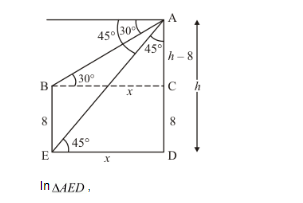# The angles of depression of the top and bottom of 8 m tall building from the

Question:

The angles of depression of the top and bottom of 8 m tall building from the top of a multistoried building are 30° and 45° respectively. Find the height of the multistoried building and the distance between the two buildings.

Solution:

Let AD be the multistoried building of height h m. And angle of depression of the top and bottom are 30° and 45°. We assume that BE = 8, CD = 8 and BC = xED = x and
AC = h − 8. Here we have to find height and distance of building.

We use trignometrical ratio.$\Rightarrow \quad \tan E=\frac{A D}{D E}$

$\Rightarrow \quad \tan 45^{\circ}=\frac{A D}{D E}$

$\Rightarrow \quad 1=\frac{h}{x}$

$\Rightarrow \quad x=h$

Again in $\triangle A B C$,

$\Rightarrow \quad \tan B=\frac{A C}{B C}$

$\Rightarrow \quad \tan 30^{\circ}=\frac{h-8}{x}$

$\Rightarrow \quad \frac{1}{\sqrt{3}}=\frac{h-8}{x}$

$\Rightarrow \quad h \sqrt{3}-8 \sqrt{3}=x$

$\Rightarrow \quad h \sqrt{3}-8 \sqrt{3}=h$

$\Rightarrow \quad h(\sqrt{3}-1)=8 \sqrt{3}$

$\Rightarrow \quad h=\frac{8 \sqrt{3}}{\sqrt{3}-1} \times \frac{\sqrt{3}+1}{\sqrt{3}+1}$

$\Rightarrow \quad h=\frac{24+8 \sqrt{3}}{2}$

$\Rightarrow \quad h=4(3+\sqrt{3})$

And

$\Rightarrow \quad x=4(3+\sqrt{3})$

Hence the required height is $4(3+\sqrt{3})$ meter and distance is $4(3+\sqrt{3})$ meter.